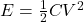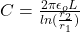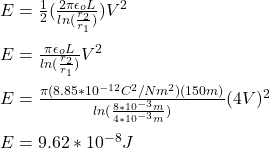## A cylindrical capacitor is made of two concentric cylinders. The inner cylinder has radius r1 = 4 mm, and the outer one a radius r2= 8 mm. T

Question

A cylindrical capacitor is made of two concentric cylinders. The inner cylinder has radius r1 = 4 mm, and the outer one a radius r2= 8 mm. The common length of the cylinders is L = 150 m. What is the potential energy stored in this capacitor when a potential difference V = 4 V is applied between the inner and outer cylinder?

in progress 0
3 weeks 2021-08-23T23:04:46+00:00 1 Answers 0 views 0

E = 9.62*10^-8 J

Explanation:

The energy stored in a capacitor is given by the following formula:(1)

E: energy stored

C: capacitance

V: potential difference of the capacitor = 4 V

The capacitance for a concentric cylindrical capacitor is:(2)

L: length of the capacitor = 150m

r2: radius of the outer cylinder  = 8mm = 8*10^-3m

r1: radius of the inner cylinder = 4mm = 4*10^-3m

εo: dielectric permittivity of vacuum = 8.85*10^-12C^2/Nm^2

You replace the expression (2) into the equation (1) and replace the values of all parameters:The energy stored in the cylindrical capacitor is 9.62*10-8 J